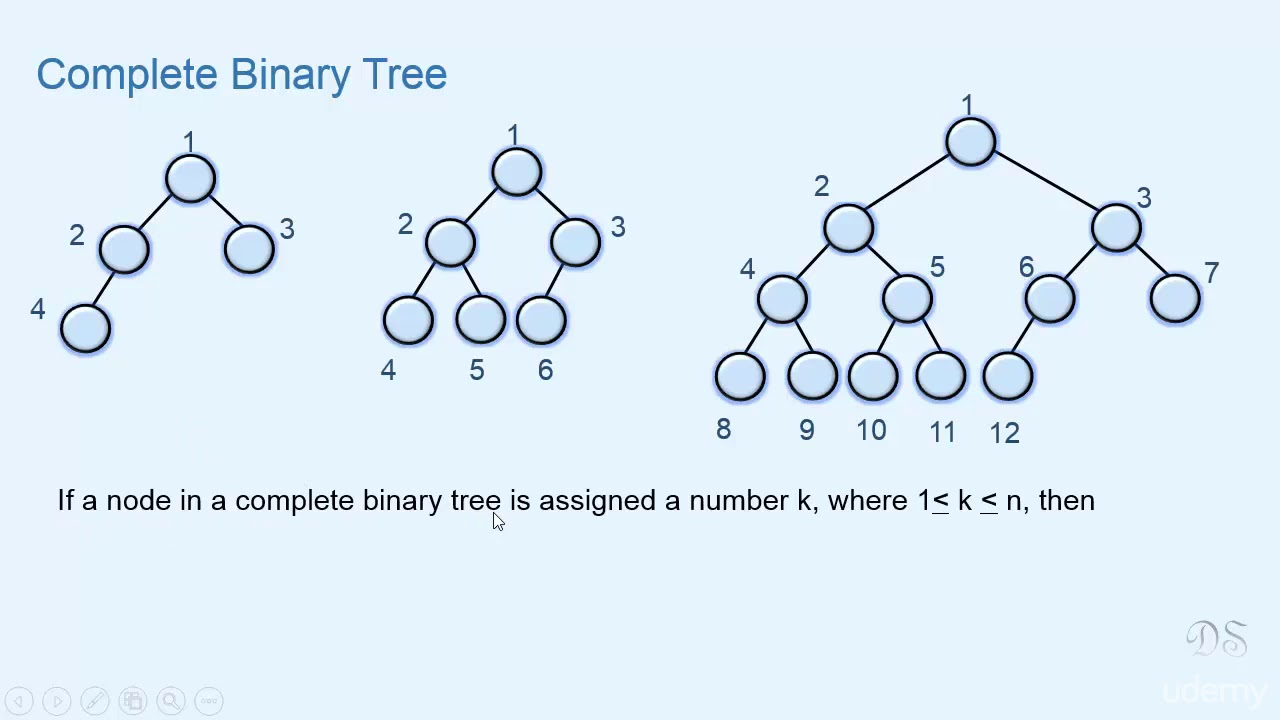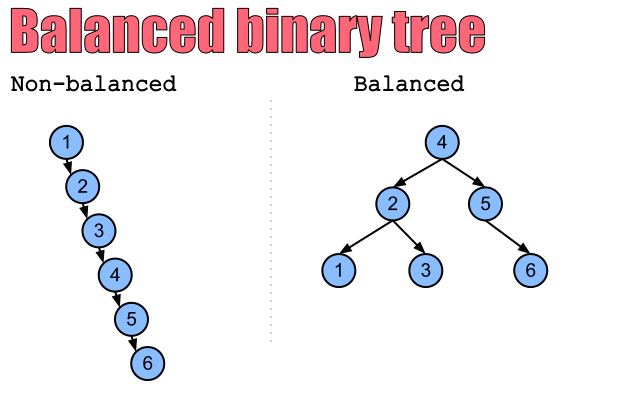# Trees Overview

### Tree​

• A data structure that consists of nodes, each with some values and pointers to child-nodes, which recursively form subtrees in the tree.
• The first node in a tree is referenced to as the root of the tree, while the nodes at the bottom of a tree (the nodes with no child-nodes) are referred to as leaf nodes or simply leaves. The paths between the root of a tree and it's leaves are called branches, and the height of a tree is the length of its longest branch. The depth of a tree node is its distance from it's tree's root; this is also known as the node's level in the tree.
• A tree is effectively a graph that's connected, directed and acyclic, that has an explicit root node, and whose nodes all have a single parent (except for the root node, which effectively has no parent). Node that in most implementations of trees, tree nodes don't have a pointer to their parent, but they can if desired.
• There are many types of trees and tree-like structures, including binary trees, heaps and tries.

### Binary Tree​

• A tree whose nodes have up to two child-nodes.
• The structure of a binary tree is such that many of it's operations have a logarithmic time complexity, making the binary tree an incredibly attractive and commonly used data structure.

### K-ary Tree​

• A tree whose nodes have upto k child-nodes. A binary tree is a k-ary tree where k == 2.

### Perfect Binary Tree​

• A binary tree whose interior nodes all have two child-nodes and whose leaf nodes all have the same depth. Example.### Complete Binary Tree​

• A binary tree that's almost perfect; its interior nodes all have two child-nodes, but it's leaf nodes don't necessarily all have the same depth. Furthermore, the nodes in the last level of a complete binary tree are far left as possible. Example:• Conversely, the following binary tree isn't complete, because the nodes in its last level aren't as far left as possible:

### Balanced Binary Tree​

• A binary tree whose nodes all have left and right subtrees whose heights differ by no more than 1.
• A balanced binary tree is such that the logarithmic time complexity of its operations is maintained.
• For example, inserting a node at the bottom of the following imbalanced binary tree's left subtree would cleary not be a logarithmic-time operation, since it would involve traversing through most of the tree's nodes:### Full Binary Tree​

A binary tree whose nodes all have either two child-nodes or zero child-nodes. Example: